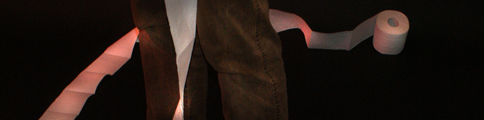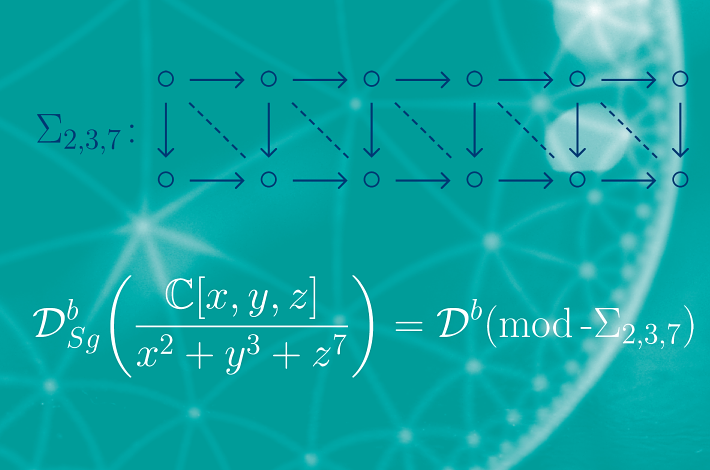Homepage of Phillip Linke - University of Bielefeld# Information on the PictureThe background consists of a triangular tiling of the hyperbolic plane whose symmetry group is the triangle group <2,3,7>. The quotient of this action is the orbifold given by the weighted projective line of type (2,3,7), thus is related to the singularity R=k[x,y,z]/(x2+y3+z7) where x, y and z have the degrees 21, 14 and 6, respectively.

Using a theorem of D. Orlov the triangulated category of singularities of R coincides with the derived category of the finite dimensional algebra Σ2,3,7 given by the depicted fully commutative quiver. Such diagrams are ubiquitous in singularity theory (cf. chapter 5 on unimodular singularities in [E]).

[E] W. Ebeling, Functions of several complex variables and their singularities, Graduate Studies in Mathematics, Vol. 83. American Mathematical Society, Providence, RI, 2007.